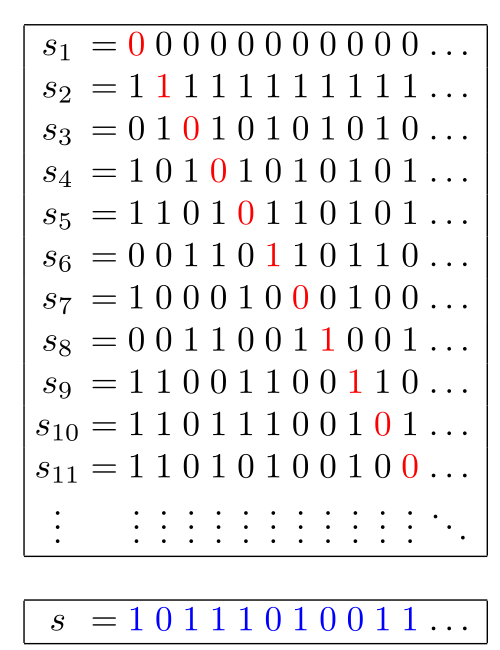# The Center of Math Blog

DO the math, DON'T overpay. We make high quality, low-cost math resources a reality.

## Thursday, July 6, 2017

### Episode 4: Uncountability of Real Numbers [#MathChops]This week’s Top Pop Math Chop comes from Georg Cantor, who first solved this piece of set theory in 1891. He presented this as a mathematical proof which showed it was impossible to link infinite sets with an infinite set of the natural numbers. This is known today as Cantor’s diagonal argument, which he proved using binary numbers.Cantor showed that if he has a list of binary numbers, takes one digit from each going diagonally, produces a new number, and swaps every single digit with a corresponding 1 or 0 (if is a 1 it becomes 0 and vice versa), that the number will be different than every other binary number listed before it. This is because in the first number the first digit is different, so it’s definitely different than the new number; in the second number the second digit is different than the second digit in the new number and so on.

You can do this same thing with real numbers, and produce infinite decimals between 0 and 1. This shows the real numbers are uncountable.

Check out the video below explaining Georg Cantor’s proof: